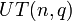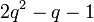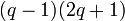# Element structure of unitriangular matrix group of degree three over a finite field

This article gives specific information, namely, element structure, about a family of groups, namely: unitriangular matrix group of degree three.
View element structure of group families | View other specific information about unitriangular matrix group of degree three

This article describes in detail the element structure of the unitriangular matrix group of degree three over a finite field. We denote the field size by$q$, the field characteristic by$p$, and the value$\log_pq$ by$r$. Further, we denote the group as$UT(3,q)$.

## Summary

Item Value
number of conjugacy classes$q^2 + q - 1$
order$q^3$
Agrees with general order formula for$UT(n,q)$:$q^{n(n-1)/2} = q^{(3)(2)/2} = q^3$
conjugacy class size statistics size 1 ($q$ times), size$q$ ($q^2 - 1$ times)
order statistics Case$p = 2$: order 1 (1 element), order 2 ($2q^2 - q - 1$ elements), order 4 ($q(q - 1)^2$ elements)
Case$p$ odd: order 1 (1 element), order$p$ ($q^3 - 1$ elements)
exponent 4 if$p = 2$$p$ if$p$ odd
The exponent depends only on$p$, not on$q$.

## Conjugacy class structure

### Number of conjugacy classes

The general theory says that number of conjugacy classes in unitriangular matrix group of fixed degree over a finite field is polynomial function of field size, where the degree of the polynomial is one less than the degree of matrices. Thus, we expect that the number of conjugacy classes is a polynomial function of$q$ of degree 3 - 1 = 2. Indeed, this is the case, and the explicit polynomial is$q^2 + q - 1$.

### Conjugacy class structure in the unitriangular matrix group

Note that the characteristic polynomial of all elements in this group is$(t - 1)^3$, hence we do not devote a column to the characteristic polynomial.

For reference, we consider matrices of the form:$\begin{pmatrix} 1 & a_{12} & a_{13} \\ 0 & 1 & a_{23} \\ 0 & 0 & 1 \\\end{pmatrix}$

Nature of conjugacy class Jordan block size decomposition Minimal polynomial Size of conjugacy class Number of such conjugacy classes Total number of elements Order of elements in each such conjugacy class Type of matrix
identity element 1 + 1 + 1 + 1$t - 1$ 1 1 1 1$a_{12} = a_{13} = a_{23} = 0$
non-identity element, but central (has Jordan blocks of size one and two respectively) 2 + 1$(t - 1)^2$ 1$q - 1$$q - 1$$p$$a_{12} = a_{23} = 0$,$a_{13} \ne 0$
non-central, has Jordan blocks of size one and two respectively 2 + 1$(t - 1)^2$$q$$2(q - 1)$$2q(q - 1)$$p$$a_{12}a_{23} = 0$, but not both$a_{12}$ and$a_{23}$ are zero
non-central, has Jordan block of size three 3$(t - 1)^3$$q$$(q - 1)^2$$q(q - 1)^2$$p$ if$p$ odd
4 if$p = 2$
both$a_{12}$ and$a_{23}$ are nonzero
Total (--) -- -- --$q^2 + q - 1$$q^3$ -- --

### Grouping by conjugacy class sizes

Conjugacy class size Total number of conjugacy classes of this size Total number of elements Cumulative number of conjugacy classes Cumulative number of elements
1$q$$q$$q$$q$$q$$q^2 - 1$$q^3 - q$$q^2 + q - 1$ (total)$q^3$ (total)

### Conjugacy classes with respect to the general linear group

If we consider the action of the general linear group by conjugation, then there is considerable fusion of conjugacy classes. Specifically, there are only three equivalence classes, corresponding to the set of unordered integer partitions of 3 describing the possible Jordan block decompositions.

Below is a summary of the information:

Jordan block size decomposition (partition of 3) Number of conjugacy classes of size 1
Number of elements in these
Number of conjugacy classes of size$q$
Number of elements in these
Total (number of conjugacy classes, elements)
1 + 1 + 1 1
1
0
0
1
1
2 + 1$q - 1$$q - 1$$2(q - 1)$$2q(q - 1)$$3(q - 1)$$(q - 1)(2q + 1)$
3 0
0$(q - 1)^2$$q(q - 1)^2$$(q - 1)^2$$q(q - 1)^2$
Total (--)$q$$q$$q^2 - 1$$q^3 - q$$q^2 + q - 1$$q^3$